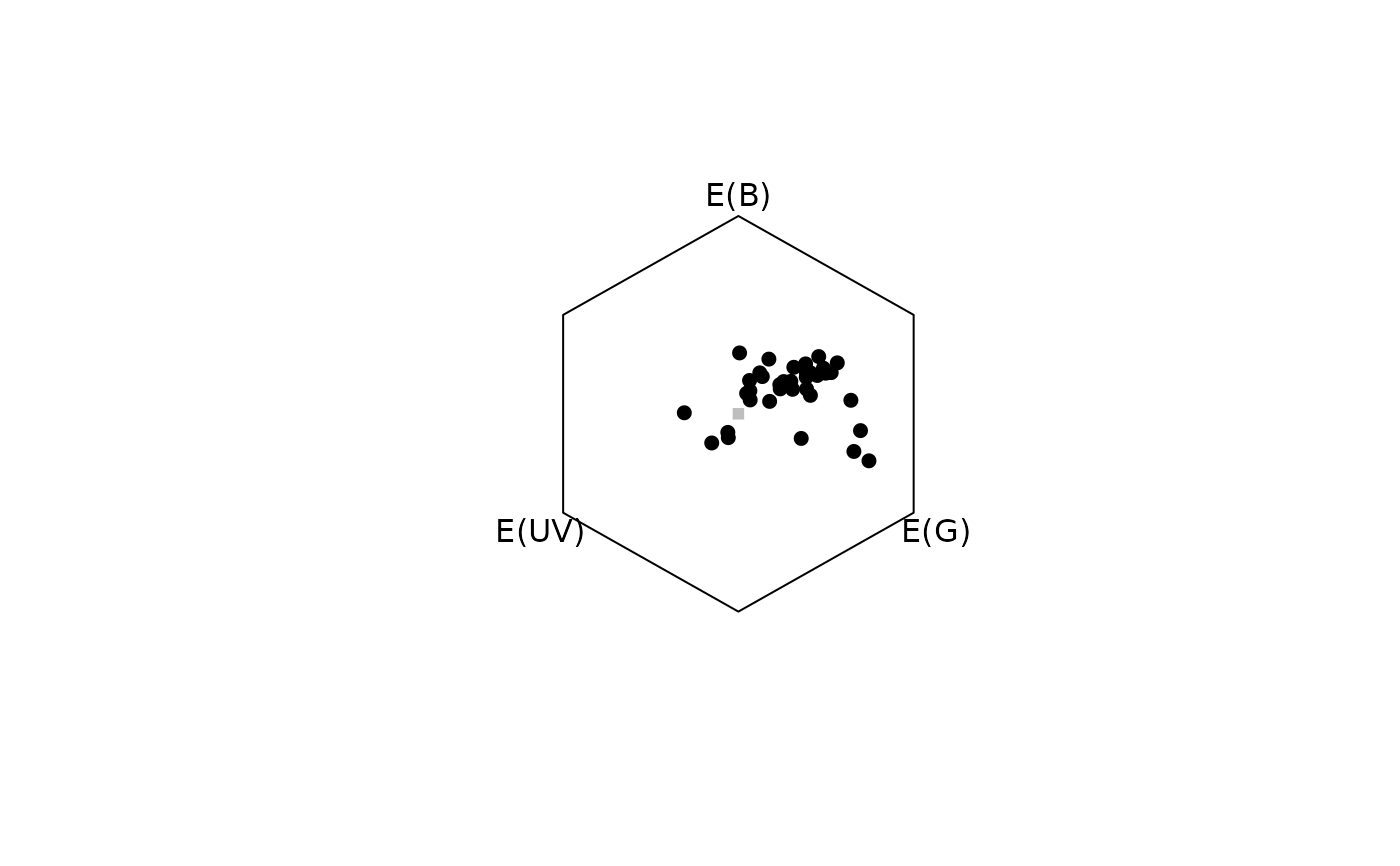Produces a colour hexagon plot.

hexplot(
hexdata,
achro = TRUE,
labels = TRUE,
sectors = c("none", "fine", "coarse"),
sec.col = "grey",
out.lwd = 1,
out.lty = 1,
out.lcol = "black",
labels.cex = 1,
achrosize = 0.8,
achrocol = "grey",
square = TRUE,
margins = NULL,
...
)

## Arguments

hexdata

(required) a data frame, possibly a result from the colspace() or hexagon() function, containing values for the 'x' and 'y' coordinates as columns (labeled as such)

achro

should a point be plotted at the origin (defaults to TRUE)?

labels

logical. Should the name of each cone be printed next to the corresponding vertex?

sectors

plot the bee-hue sector dividers? Options are:

• "none": No sectors (default)

• "fine": 36 10-degree sectors

• "coarse": six bee-hue sectors (blue, blue-green, green, uv-green, uv, uv-blue).

sec.col

line colour of hue sector dividers. Defaults to "grey".

out.lwd

graphical parameters for the plot outline.

out.lty

graphical parameters for the plot outline.

out.lcol

graphical parameters for the plot outline.

labels.cex

size of the arrow labels.

achrosize

size of the point at the origin when achro = TRUE (defaults to 0.8).

achrocol

color of the point at the origin achro = TRUE (defaults to 'grey').

square

logical. Should the aspect ratio of the plot be held to 1:1? (defaults to TRUE).

margins

Deprecated. Please use the standard par() method for custom margins.

...

additional graphical options. See par().

## Author

Thomas White thomas.white026@gmail.com

## Examples

data(flowers)
vis.flowers <- vismodel(flowers,
visual = "apis", qcatch = "Ei", relative = FALSE,
vonkries = TRUE, achromatic = "l", bkg = "green"
)
hex.flowers <- colspace(vis.flowers, space = "hexagon")
plot(hex.flowers)